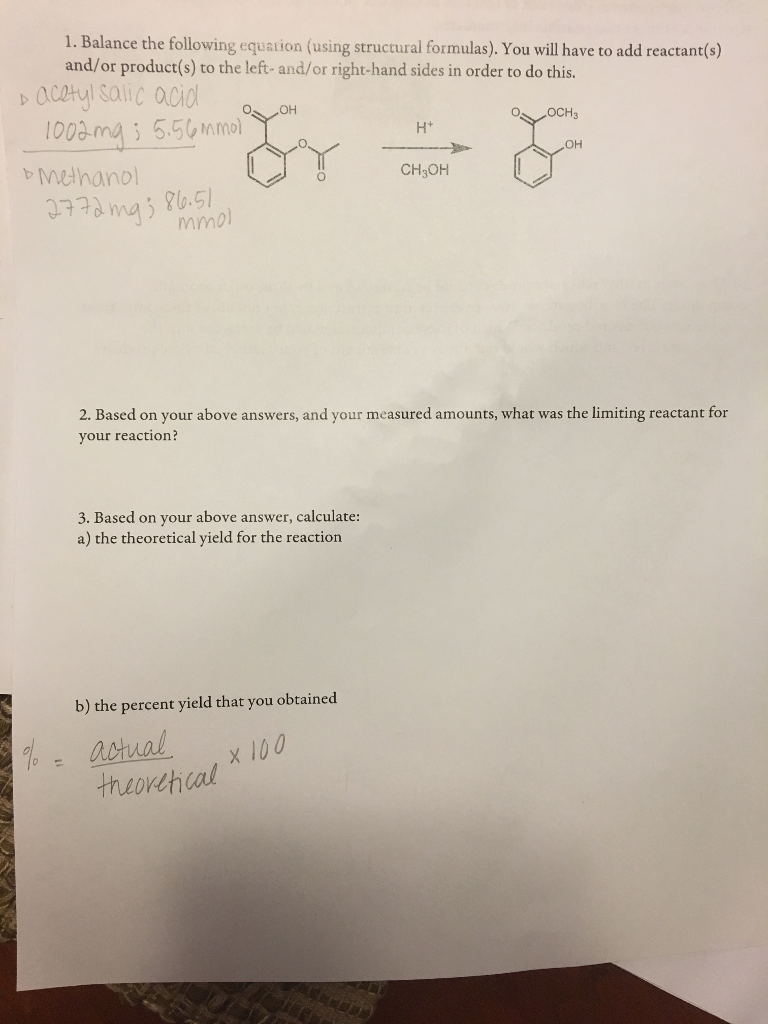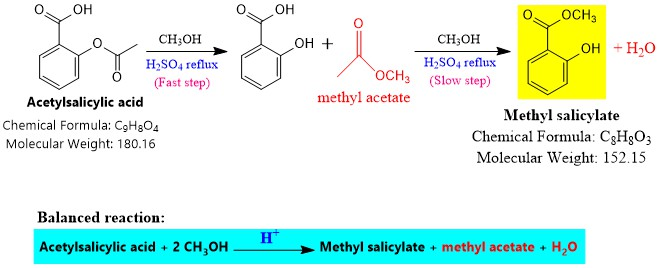# Question & Answer: Balance the following equation (using structural formulas). You will have to add reactant(s)…..Balance the following equation (using structural formulas). You will have to add reactant(s) and/or product(s) to the left- and/or right-hand sides in order to do this. Based on your above answers, and your measured amounts, what was the limiting reactant for your reaction? Based on your above answer, calculate: a) the theoretical yield for the reaction b) the percent yield that you obtained.

Ans. #1. See the figure.

Don't use plagiarized sources. Get Your Custom Essay on
Question & Answer: Balance the following equation (using structural formulas). You will have to add reactant(s)…..
GET AN ESSAY WRITTEN FOR YOU FROM AS LOW AS \$13/PAGE#2. See the balanced reaction, 1 mol acetyl salicylic acid reacts with 2 mol methanol to produce a mol each of methyl salicylate, methyl acetate and water.

Theoretical molar ration of reactants is- acetyl salicylic acid : methanol = 1 : 2

Given,

Moles of acetyl salicylic acid = 5.56 millimoles

Mole of methanol = 86.51 millimoles

Now,

Experimental molar ration of reactants is- acetyl salicylic acid : methanol

= 5.56 : 86.51 = 1 : 15.56

Compare the theoretical and experimental molar ratio of reactants, methanol is present is excess. Thus, acetyl salicylic acid is the limiting reactant.

#3. A. See the balanced reaction, 1 mol acetyl salicylic acid produces 1 mol methyl salicylate.

Theoretically, 5.56 mmol acetyl salicylic acid shall produce 5.56 mmoles of methyl salicylate, too.

So,

Theoretical moles of methyl salicylate produced = 5.56 mmoles = 0.00556 moles

Theoretical yield = Theoretical moles produced x molar mass

= 0.00556 mol x (152.15 g/mol)

0.846 g

#3.B. % yield = (Actual yield/ Theoretical yield) x 100

= (Actual yield/ 0.846 g) x 100

Put the actual yield (mass) you obtained experimentally in above reaction to get the exact value.